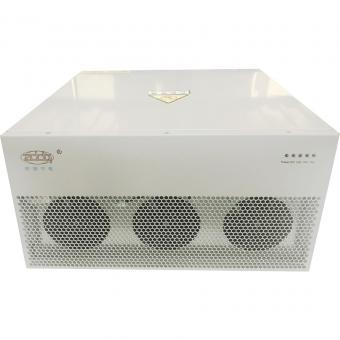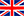English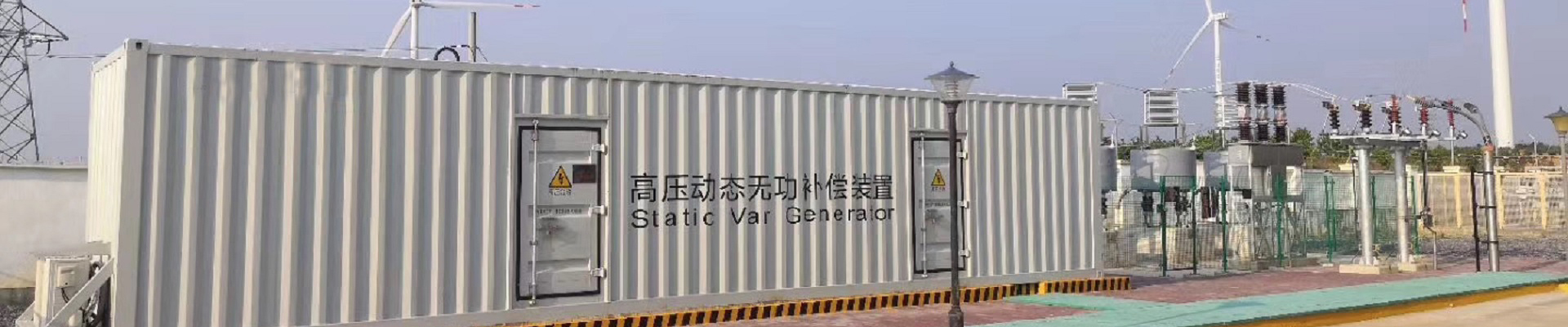Active Harmonic filter, a power correction system to mitigate harmonics and reduce voltage flucturation and then enhance network reliability, boost system capacity,reduce operating expenses and improve power quality of power grid.

•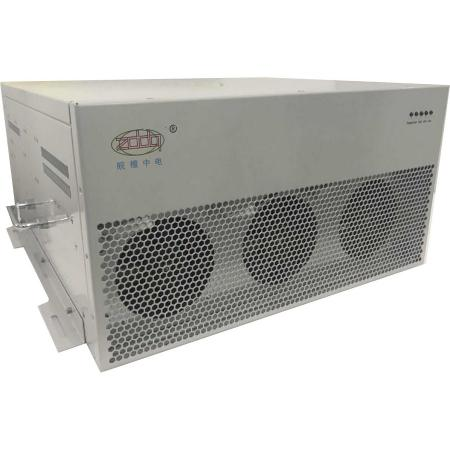#### Power quality conditioner (Active harmonic filter)

Power quality conditioner (Active harmonic filter/AHF) is connected in parallel with non-linear loads, and uses one set of CT to detect the load current. It calculates each order harmonic current by FFT algorithms in its DSP microchips, and then generates a compensating current with the same amplitude but opposite phase angles to the detected harmonic current, which cancels out the original load harmonics.Not only eliminates harmonic current from the load side, but it also mitigates harmonic voltage caused by harmonic currents. The AHF system can also improve power factor (PF) and correct load imbalances in the power system.
•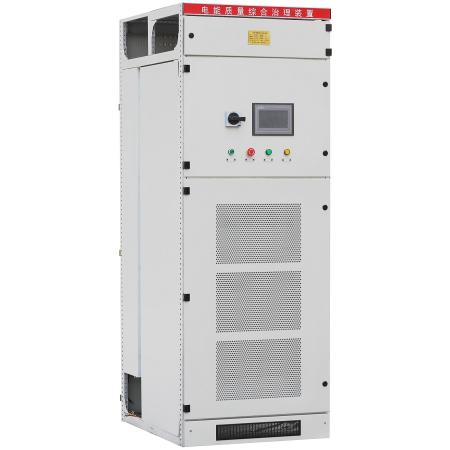#### Metal enclosed harmonic filter banks

Active harmonic filter is connected in parallel with non-linear loads, and uses one set of CT to detect the load current. It calculates each order harmonic current by FFT algorithms in its DSP microchips, and then generates a compensating current with the same amplitude but opposite phase angles to the detected harmonic current, which cancels out the original load harmonics.Not only eliminates harmonic current from the load side, but it also mitigates harmonic voltage caused by harmonic currents. The AHF system can also improve power factor (PF) and correct load imbalances in the power system.
•#### active harmonic filter for harmonics reduction

Active harmonic filter is connected in parallel with non-linear loads, and uses one set of CT to detect the load current. It calculates each order harmonic current by FFT algorithms in its DSP microchips, and then generates a compensating current with the same amplitude but opposite phase angles to the detected harmonic current, which cancels out the original load harmonics.Not only eliminates harmonic current from the load side, but it also mitigates harmonic voltage caused by harmonic currents. The AHF system can also improve power factor (PF) and correct load imbalances in the power system.
•#### Active harmonic analysis Filtering system

Active harmonic filter is connected in parallel with non-linear loads, and uses one set of CT to detect the load current. It calculates each order harmonic current by FFT algorithms in its DSP microchips, and then generates a compensating current with the same amplitude but opposite phase angles to the detected harmonic current, which cancels out the original load harmonics.Not only eliminates harmonic current from the load side, but it also mitigates harmonic voltage caused by harmonic currents. The AHF system can also improve power factor (PF) and correct load imbalances in the power system.
•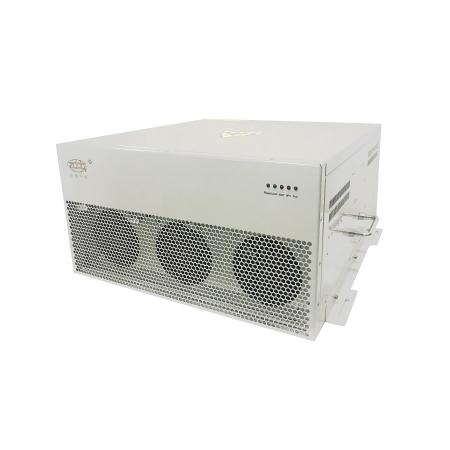#### Advanced Active Power Filter AAPF

Advanced Active Power Filter AAPF is connected in parallel with non-linear loads, and uses one set of CT to detect the load current. It calculates each order harmonic current by FFT algorithms in its DSP microchips, and then generates a compensating current with the same amplitude but opposite phase angles to the detected harmonic current, which cancels out the original load harmonics.APF not only eliminates harmonic current from the load side, but it also mitigates harmonic voltage caused by harmonic currents. The APF system can also improve power factor (PF) and correct load imbalances in the power system.
•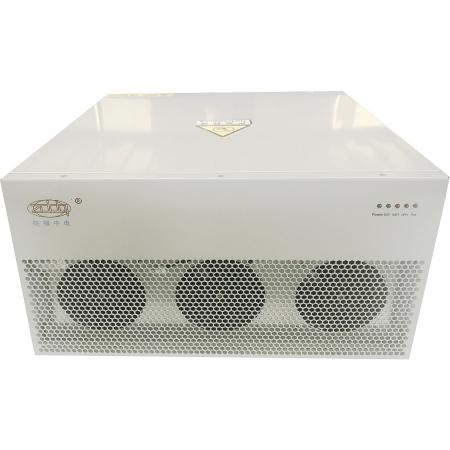#### 25A Active Power Filter

Active Power filter(APF) is connected in parallel with non-linear loads, and uses one set of CT to detect the load current. It calculates each order harmonic current by FFT algorithms in its DSP microchips, and then generates a compensating current with the same amplitude but opposite phase angles to the detected harmonic current, which cancels out the original load harmonics.Not only eliminates harmonic current from the load side, but it also mitigates harmonic voltage caused by harmonic currents. The AHF system can also improve power factor (PF) and correct load imbalances in the power system.
1 2 3 4 5 6

a total of 6 pages

Hot Products
•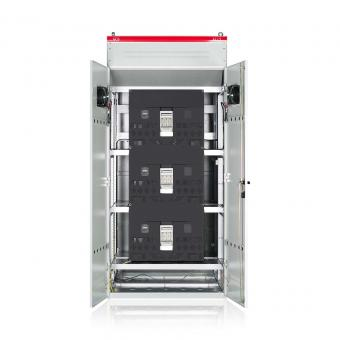#### Capacitor banks and harmonic filters. Low voltage

•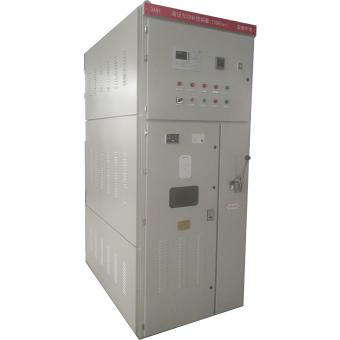#### 3.3KV Local reactive power compensation for motors,Arc Furnace

•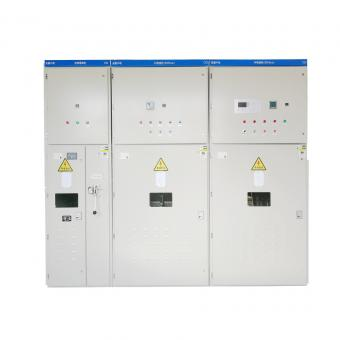#### Medium voltage Automatic Capacitor banks with harmonic filters

•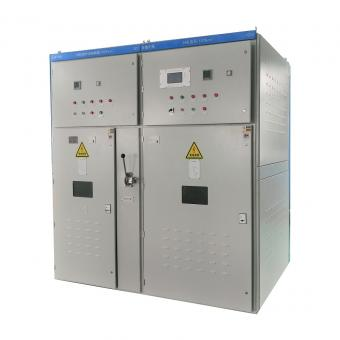#### High voltage Automatic Power Factor improvement plant for Motors VFDs

•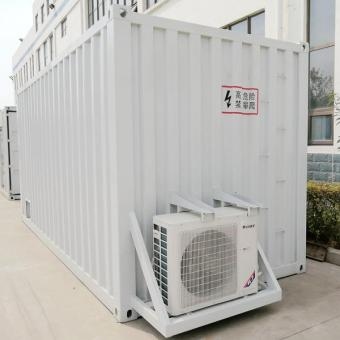#### High voltage static var generator HV SVG Statcom outdoor container

•#### Medium voltage outdoor Static Synchronous Compensator(STATCOM)

•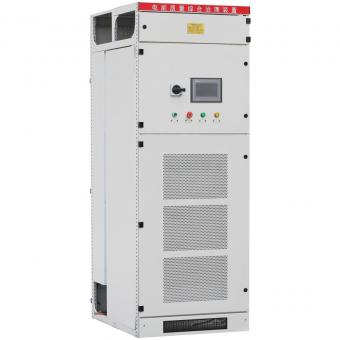•